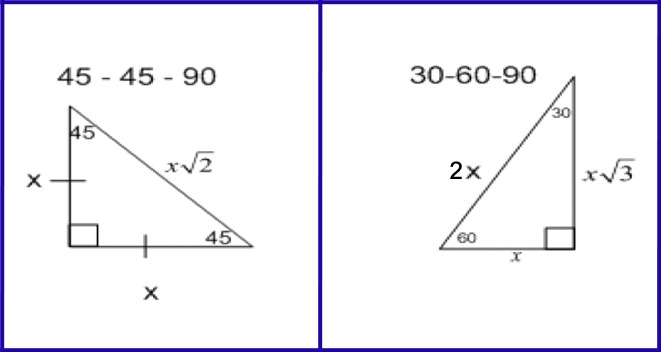Special Right Triangles
4 years ago
cbusch
Save
Edit
Host a game
Live GameLive
Homework
Solo Practice
Practice10 QuestionsShow answers
• Question 1
180 seconds
Q. A square has side length 95. What is the length of the diagonal of the square? Express your answer in simplest radical form.
134.4
67.2
95√2
(95√2)/2
• Question 2
180 seconds
Q. A square has diagonal length 13 m. What is the side length of the square, to the nearest centimeter?
9.19
18.38
5.10
13
• Question 3
180 seconds
Q. An equilateral triangle has height 26 cm. What is the length of each side of the triangle, to the nearest centimeter?
26, 13, 23
26, 26, 38
26, 15, 30
26, 42, 38
• Question 4
60 secondsQ. Find the value of y.
8
4
2√3
8√3
• Question 5
60 secondsQ. Find the value of y to the nearest tenth.
4.5
3.2
2.3
5
• Question 6
60 secondsQ. Find the value of y.
7
7√3
14√3
(14√3)/3
• Question 7
60 secondsQ. Find the value of y.
11√2
11
22
(11√2)/2
• Question 8
60 secondsQ. Find the value of x.
18
18√3
36√3
12
• Question 9
60 secondsQ. Find the value of y.
9
18√2
9√2
(9√2)/2
• Question 10
180 seconds
Q. A square tablecloth has a line of embroidered flowers along the diagonal. The tablecloth is 48 in. on each side. How long is the embroidery line?  Round to the nearest inch.